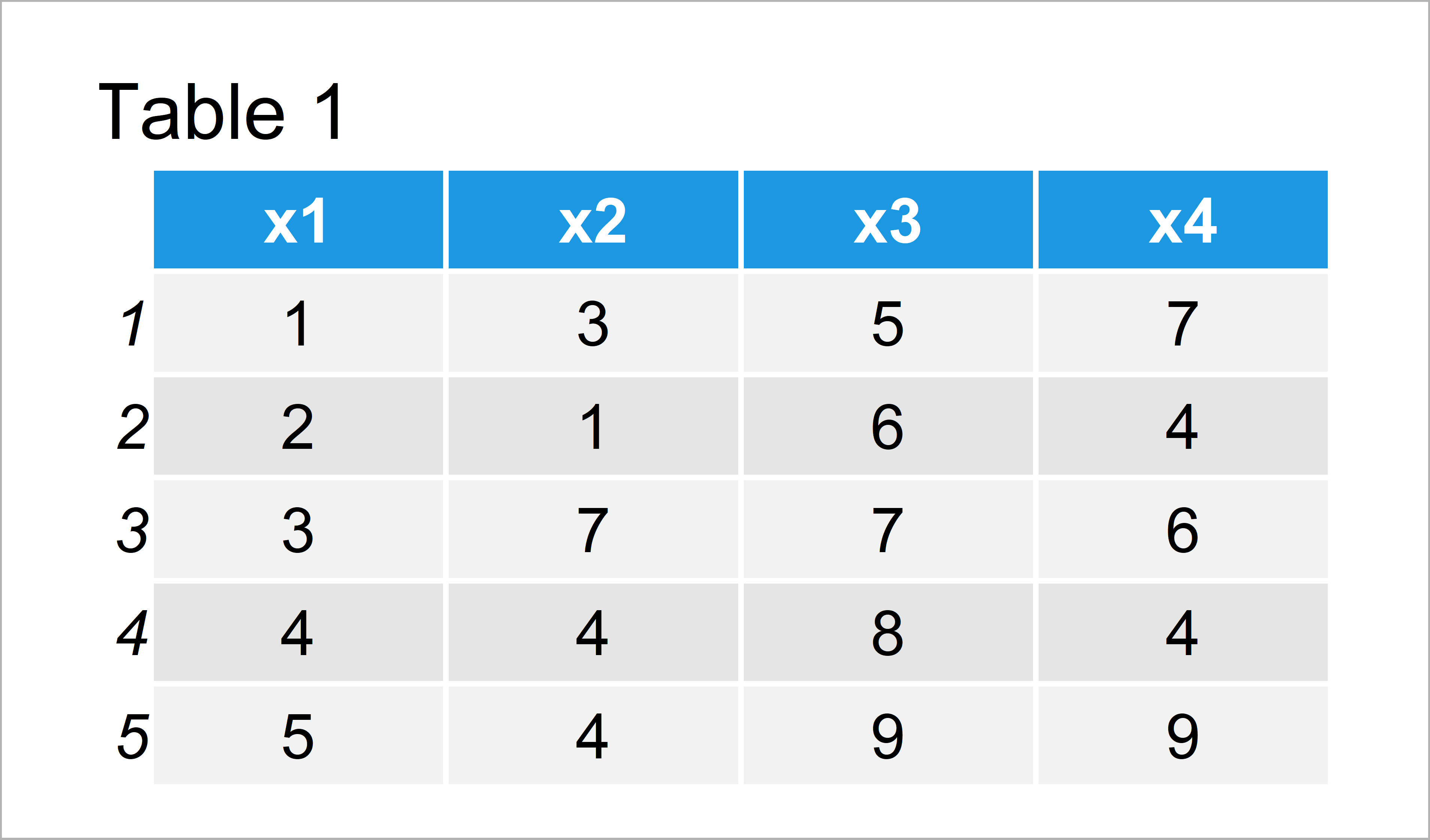# Sum of Two or Multiple Data Frame Columns in R (2 Examples)

In this article you’ll learn how to compute the sum across two or more columns of a data frame in the R programming language.

Let’s dive into it!

## Example Data

The first step is to define some example data:

```data <- data.frame(x1 = 1:5,             # Create data frame
x2 = c(3, 1, 7, 4, 4),
x3 = 5:9,
x4 = c(7, 4, 6, 4, 9))
data                                     # Print data frame```As you can see based on Table 1, our example data is a data frame consisting of five rows and four columns. All the variables are numeric.

## Example 1: Calculate Sum of Two Columns Using + Operator

In this example, I’ll explain how to get the sum across two columns of our data frame.

For this, we can use the + and the \$ operators as shown below:

```data\$x1 + data\$x2                        # Sum of two columns
#   4  3 10  8  9```

After executing the previous R code, the result is shown in the RStudio console.

## Example 2: Calculate Sum of Multiple Columns Using rowSums() & c() Functions

It is also possible to return the sum of more than two variables. To efficiently calculate the sum of the rows of a data frame subset, we can use the rowSums function as shown below:

```rowSums(data[ , c("x1", "x2", "x4")])    # Sum of multiple columns
#  11  7 16 12 18```

The result of the addition of the variables x1, x2, and x4 is shown in the RStudio console.

## Video, Further Resources & Summary

Do you want to learn more about sums and data frames in R? Then I recommend having a look at the following video of my YouTube channel. I show the R code of this tutorial in the video:

Please accept YouTube cookies to play this video. By accepting you will be accessing content from YouTube, a service provided by an external third party.If you accept this notice, your choice will be saved and the page will refresh.

Also, you might read the other articles on this website.